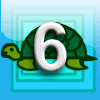#### You may also like### Special Numbers

My two digit number is special because adding the sum of its digits to the product of its digits gives me my original number. What could my number be?### Your Number Is...

Think of a number and follow the machine's instructions... I know what your number is! Can you explain how I know?### First Forward Into Logo 6: Variables and Procedures

Learn to write procedures and build them into Logo programs. Learn to use variables.

# Perimeter Expressions

### Why do this problem?

This problem gives students plenty of opportunity to practise manipulating algebraic expressions within a purposeful context. Along the way, the challenges will provoke some insights that will be worth sharing. Issues relating to the dimensions in formulae for areas and lengths might emerge.

### Possible approach

This printable worksheet may be useful: Perimeter Expressions.pdf

The worksheet is also available in French: Expressions de pÃ©rimÃ¨tres
We are grateful to Clara Huizink for translating the original.

Hand out sheets of paper (A4 is ideal) and introduce the problem, giving students time to cut out their five rectangles.

Show the image of Charlie's shape and ask students to work out the perimeter in terms of $a$ and $b$. The area of the smallest rectangle could be designated $R$ and used as a unit to express other areas, if students are not confident enough in their algebra to express it in terms of $a$ and $b$.

Once everyone is happy that the area is $9R$ or $9ab$ and the perimeter is $10a + 4b$, ask them to combine the largest and smallest rectangles (edge to edge, corner to corner) to make other shapes, and work out the areas and perimeters. How many different perimeters can they find?

Once they have done this, discuss: are they surprised that they only found one other possible perimeter, and that the two answers could be reached in several different ways?

The next challenge is to make different shapes using at least two of the rectangles, and again work out the area and perimeter. Students could make a shape in pairs and work out the area and perimeter to give to another pair (without letting them see the shape), to see if they can create a shape with the same area and perimeter. There is opportunity for fruitful discussion when the two shapes are compared, to see what is the same and what is different, and to see if one can be transformed into the other.

Alternatively, collect together the areas and perimeters on the board. Then in pairs, challenge students to combine rectangles to make each of the area/perimeter combinations listed on the board, checking each other's work as they go along.

Finally, the "questions to consider" provide some interesting points for discussion - students could be invited to suggest questions they think mathematicians might ask themselves, with the list in the problem used to prompt ideas if necessary.

### Key questions

How do we know whether an expression represents an area rather than a perimeter?

What does the area expression tell us about the pieces used to make the shape?
Are there multiple ways to make a given area/perimeter combination?

### Possible extension

Number Pyramids builds on this activity by challenging students to create algebraic expressions to explain a relationship.

Fit for Photocopying is a very challenging extension, involving exploration of ratio and functions in the context of paper sizes.

### Possible support

Use numerical values, instead of $a$ and $b$, and cut the rectangles out from squared paper. Begin by looking for numerical relationships rather than algebraic ones, and perhaps introduce the algebra to explain the patterns students find.